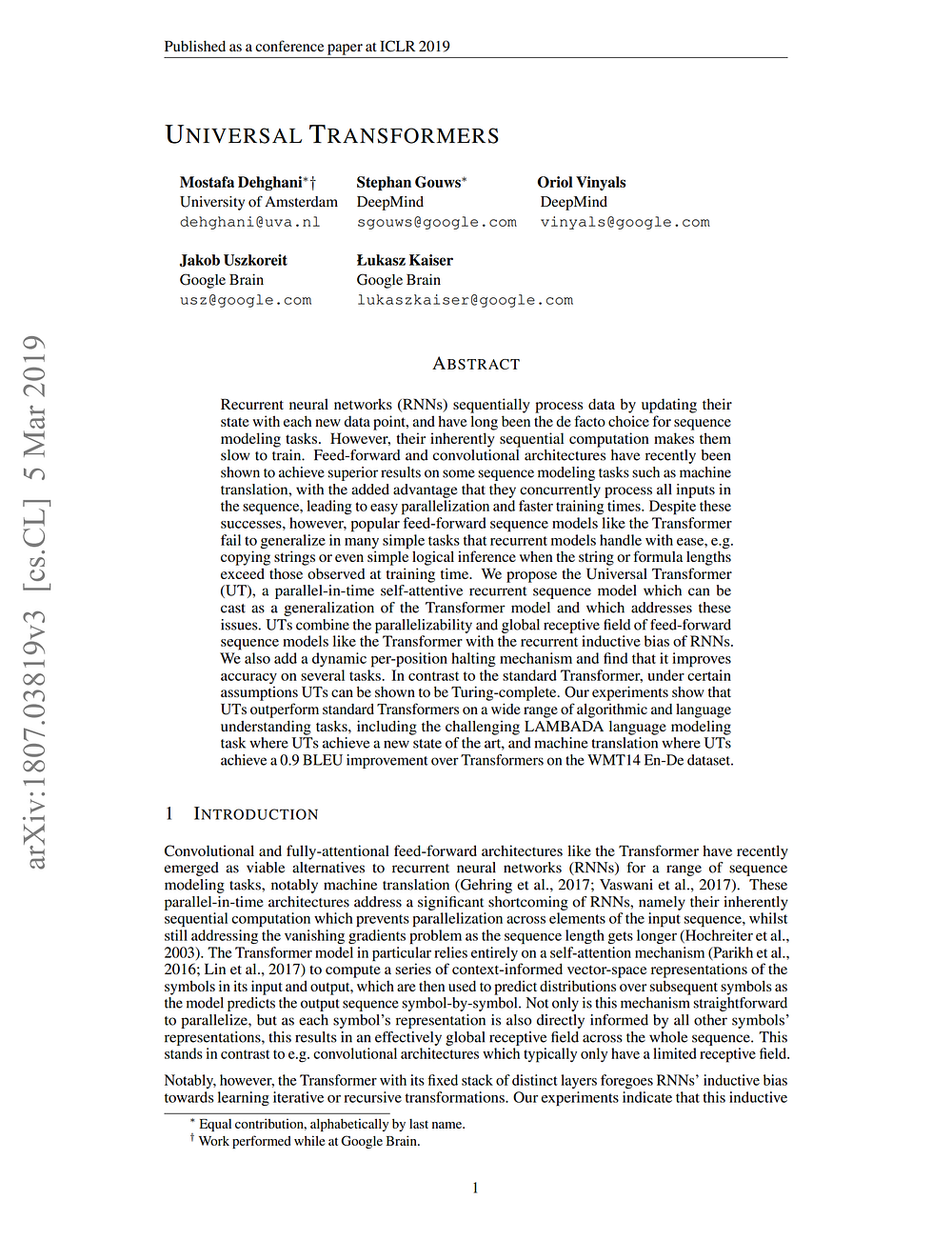# Adaptive Computation Time (ACT) in Neural Networks [3/3]

Source: Deep Learning on Medium## Turing completeness

The interesting aspect of UT is its universality. Under certain assumptions, the resulting Universal Transformer can be shown to be Turing-complete (or “computationally universal”).

Remember, RNNs are considered Turing complete (here is a proof by Hava Siegelmann and Eduardo Sonntag). Yet there are controversies and statements that practically this is not true.

Regarding the original Transformer, assuming finite precision, it can be shown that the Transformer cannot be computationally universal (yet there are controversies as well).

While the Transformer executes a total number of operations that scales with the input size, the number of sequential operations is constant and independent of the input size, determined solely by the number of layers.

An intuitive example from the author’s blog are functions whose execution requires the sequential processing of each input element. In this case, for any given choice of depth T, one can construct an input sequence of length N > T that cannot be processed correctly by a Transformer: